Indirect questions - Incorrect observation

Chapter 13 Class 11 Statistics
Concept wise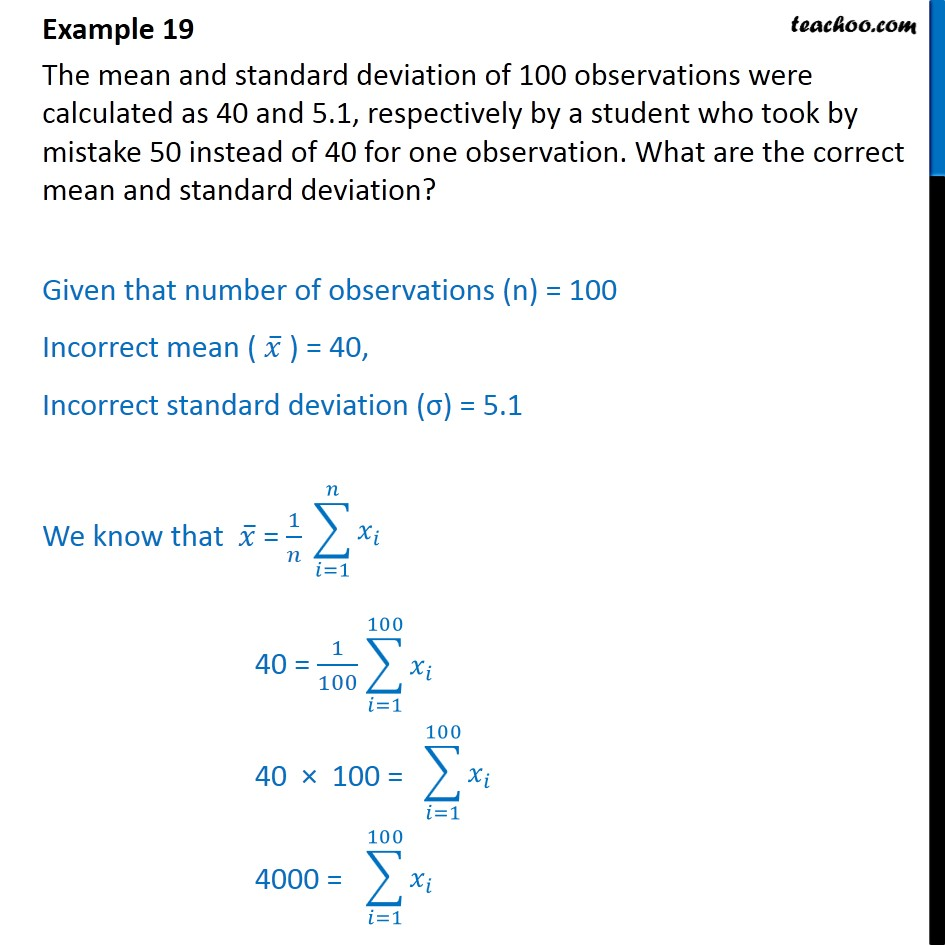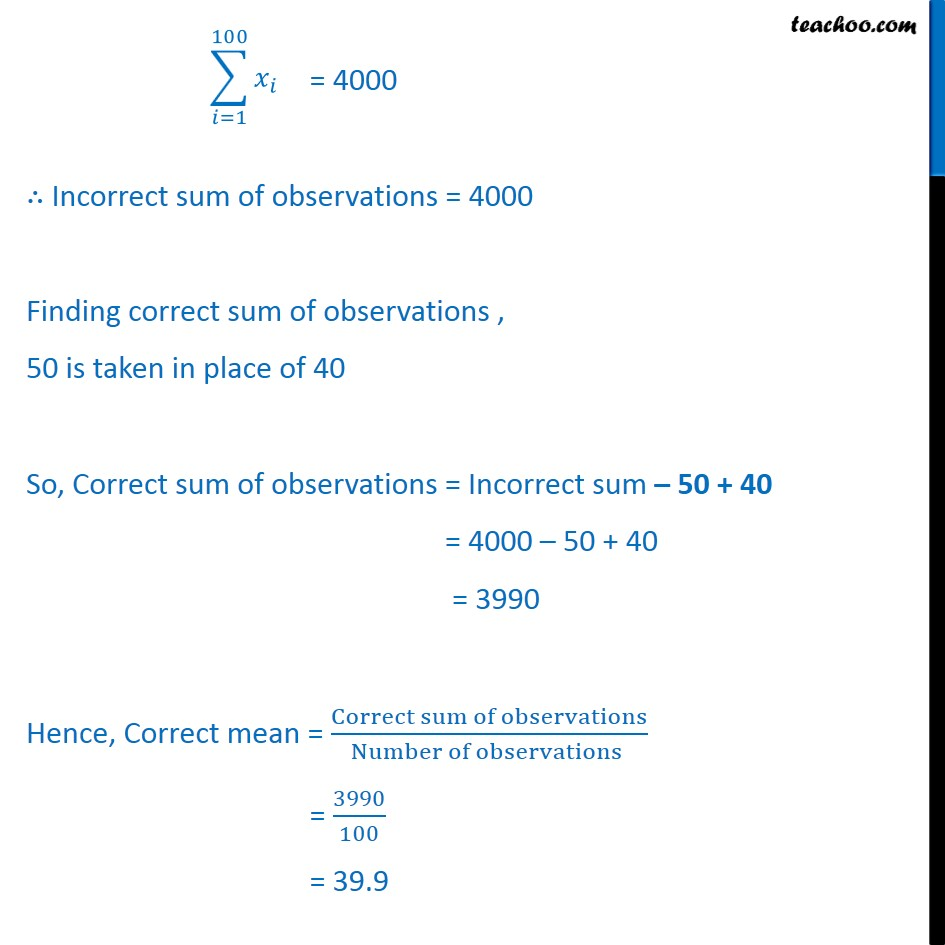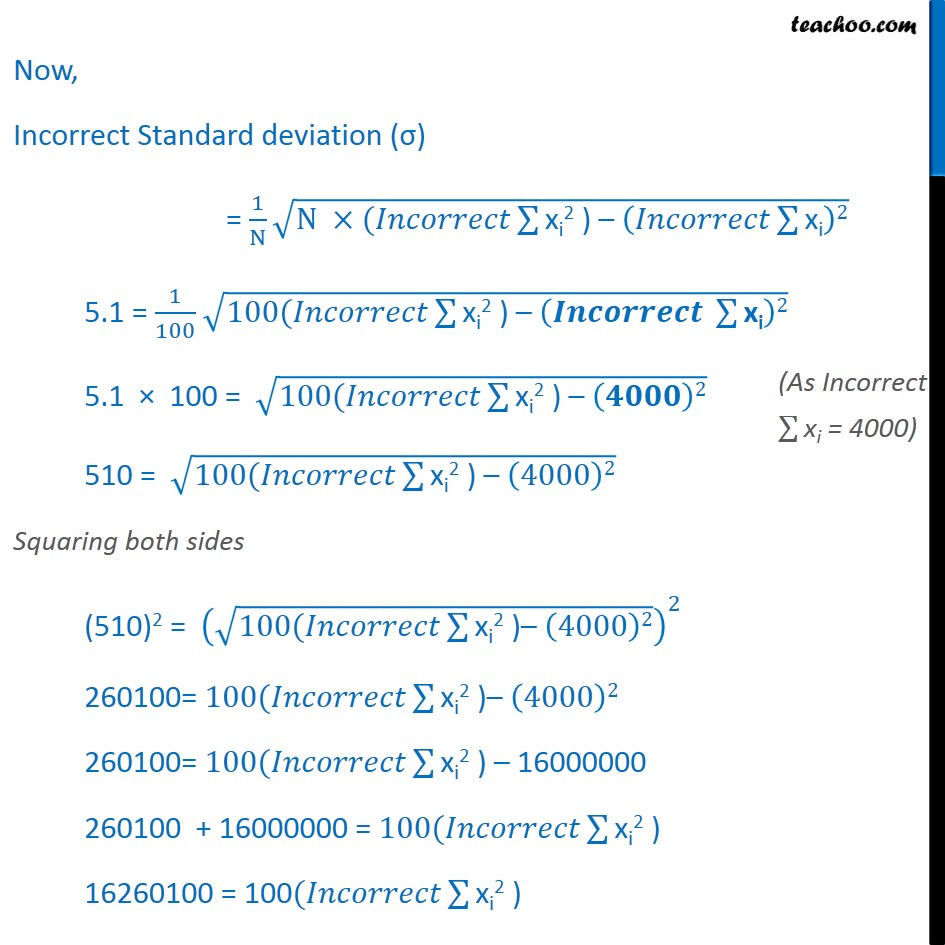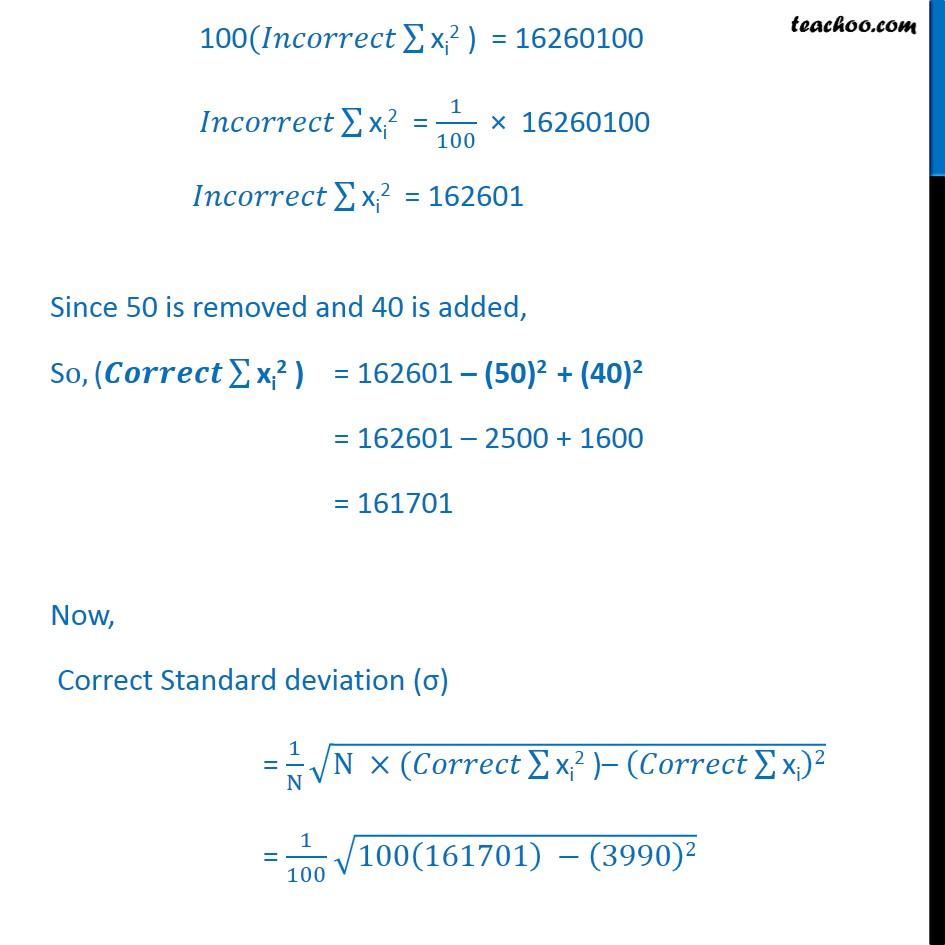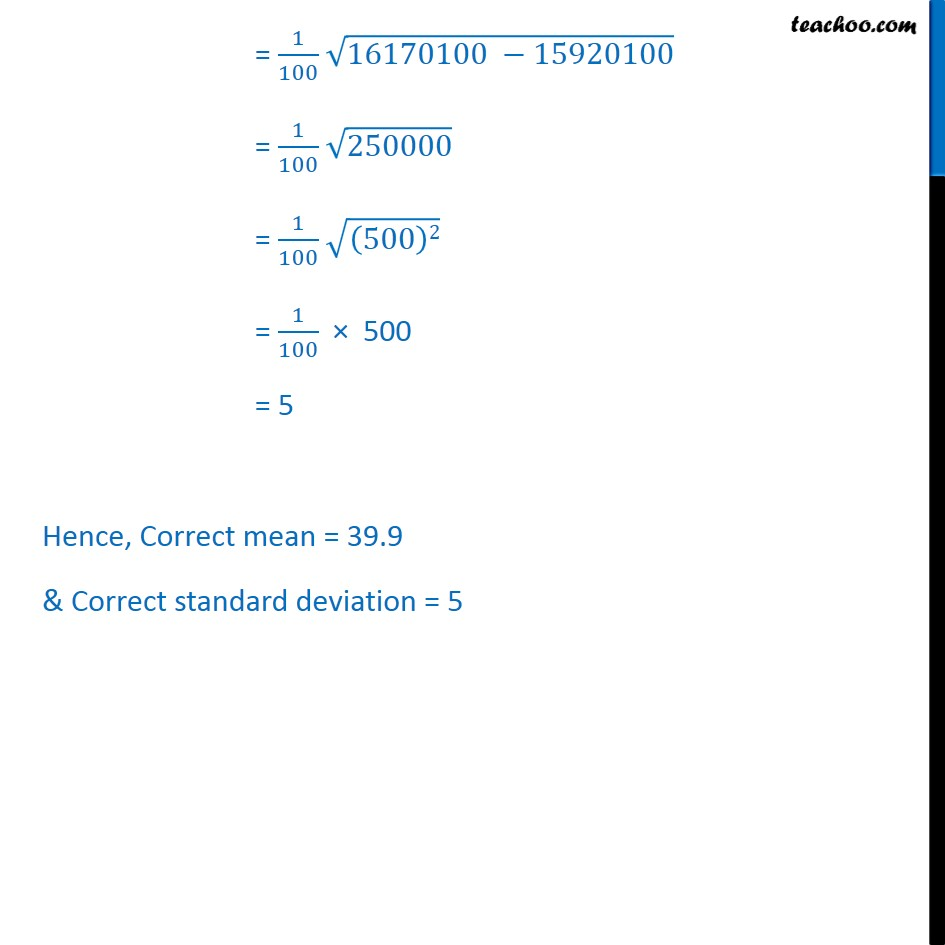Learn in your speed, with individual attention - Teachoo Maths 1-on-1 Class

### Transcript

Example 16 The mean and standard deviation of 100 observations were calculated as 40 and 5.1, respectively by a student who took by mistake 50 instead of 40 for one observation. What are the correct mean and standard deviation? Given that number of observations (n) = 100 Incorrect mean ( 𝑥﷯ ) = 40, Incorrect standard deviation (σ) = 5.1 We know that 𝑥﷯ = 1﷮𝑛﷯ 40 = 1﷮100﷯ 40 × 100 = 4000 = = 4000 ∴ Incorrect sum of observations = 4000 Finding correct sum of observations , 50 is taken in place of 40 So, Correct sum of observations = Incorrect sum – 50 + 40 = 4000 – 50 + 40 = 3990 Hence, Correct mean = Correct sum of observations﷮Number of observations ﷯ = 3990﷮100﷯ = 39.9 Now, Incorrect Standard deviation (σ) = 1﷮N﷯ ﷮N ×(𝐼𝑛𝑐𝑜𝑟𝑟𝑒𝑐𝑡 ﷮﷮xi2 ) – 𝐼𝑛𝑐𝑜𝑟𝑟𝑒𝑐𝑡 ﷮﷮xi﷯﷯2﷯﷯ 5.1 = 1﷮100﷯ ﷮100(𝐼𝑛𝑐𝑜𝑟𝑟𝑒𝑐𝑡 ﷮﷮xi2 ) – 𝑰𝒏𝒄𝒐𝒓𝒓𝒆𝒄𝒕 ﷮﷮xi﷯﷯2﷯﷯ 5.1 × 100 = ﷮100(𝐼𝑛𝑐𝑜𝑟𝑟𝑒𝑐𝑡 ﷮﷮xi2 ) – 𝟒𝟎𝟎𝟎﷯2﷯﷯ 510 = ﷮100(𝐼𝑛𝑐𝑜𝑟𝑟𝑒𝑐𝑡 ﷮﷮xi2 ) – 4000﷯2﷯﷯ Squaring both sides (510)2 = ﷮100(𝐼𝑛𝑐𝑜𝑟𝑟𝑒𝑐𝑡 ﷮﷮xi2 )– 4000﷯2﷯﷯﷯﷮2﷯ 260100= 100(𝐼𝑛𝑐𝑜𝑟𝑟𝑒𝑐𝑡 ﷮﷮xi2 )– 4000﷯2﷯ 260100= 100(𝐼𝑛𝑐𝑜𝑟𝑟𝑒𝑐𝑡 ﷮﷮xi2 ) –﷯ 16000000 260100 + 16000000 = 100(𝐼𝑛𝑐𝑜𝑟𝑟𝑒𝑐𝑡 ﷮﷮xi2 ) ﷯ 16260100 = 100(𝐼𝑛𝑐𝑜𝑟𝑟𝑒𝑐𝑡 ﷮﷮xi2 ) ﷯ 100(𝐼𝑛𝑐𝑜𝑟𝑟𝑒𝑐𝑡 ﷮﷮xi2 ) ﷯ = 16260100 𝐼𝑛𝑐𝑜𝑟𝑟𝑒𝑐𝑡 ﷮﷮xi2 ﷯ = 1﷮100﷯ × 16260100 𝐼𝑛𝑐𝑜𝑟𝑟𝑒𝑐𝑡 ﷮﷮xi2 ﷯ = 162601 Since 50 is removed and 40 is added, So, (𝑪𝒐𝒓𝒓𝒆𝒄𝒕 ﷮﷮xi2 ) ﷯ = 162601 – (50)2 + (40)2 = 162601 – 2500 + 1600 = 161701 Now, Correct Standard deviation (σ) = 1﷮N﷯ ﷮N ×(𝐶𝑜𝑟𝑟𝑒𝑐𝑡 ﷮﷮xi2 )– 𝐶𝑜𝑟𝑟𝑒𝑐𝑡 ﷮﷮xi﷯﷯2﷯﷯ = 1﷮100﷯ ﷮100 161701﷯ − 3990﷯2﷯ = 1﷮100﷯ ﷮16170100 −15920100﷯ = 1﷮100﷯ ﷮250000﷯ = 1﷮100﷯ ﷮ 500﷯2﷯ = 1﷮100﷯ × 500 = 5 Hence, Correct mean = 39.9 & Correct standard deviation = 5